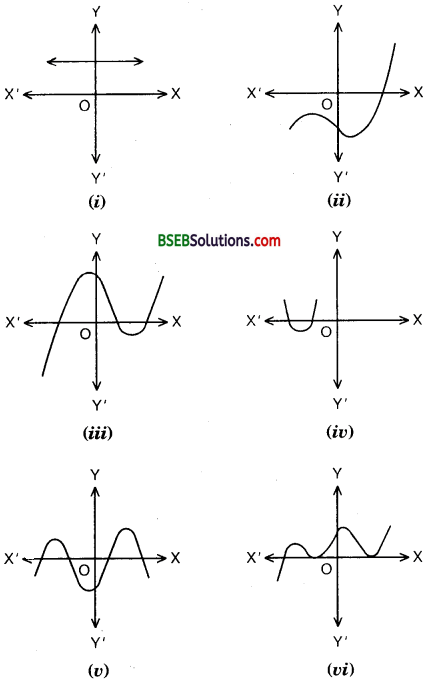# Bihar Board Class 10th Maths Solutions Chapter 2 Polynomials Ex 2.1

Bihar Board Class 10th Maths Solutions Chapter 2 Polynomials Ex 2.1 Textbook Questions and Answers.

## BSEB Bihar Board Class 10th Maths Solutions Chapter 2 Polynomials Ex 2.1

Question 1.
The graphs of y = p(x) are given in figures below for some polynomials p(x). Find the number of zeroes of p(x), in each case.Solution:

1. There are no zeros as the graph does not intersect the x-axis.
2. The number of zeroes is one as the graph intersects the x-axis at one point only.
3. The number of zeroes is three as the graph intersects the x-axis at three points.
4. The number of zeroes is two as the graph intersects the x-axis at two points.
5. The number of zeroes is four as the graph intersects the x-axis at four points.
6. The number of zeroes is three as the graph intersects the x-axis three points.